## 4 Colors, Ratio of Color Frequencies is 2:2:4, One-sided

If three out of four colors are used for each piece we can divide the whole set of 648 pieces into four subsets with one out of four colors missing. If you take these subsets of 162 pieces each you can solve four figures, which fit together to get a figure with the whole set. As an example a 18x36 rectangle made of four 9x18 rectangles is shown.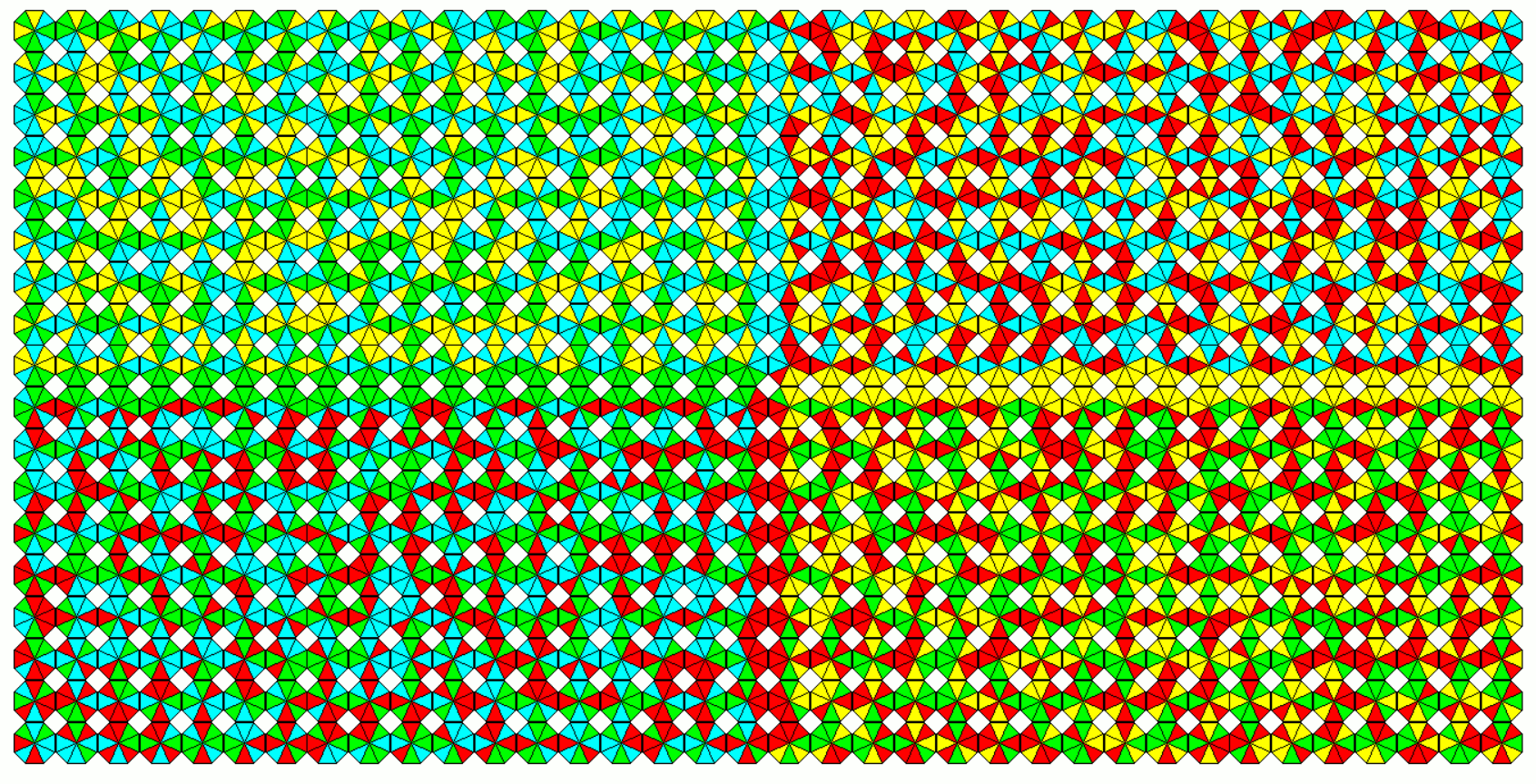## 4 Colors, Ratio of Color Frequencies is 2:2:4, Two-sided

There are 396 two-sided pieces shown as a rectangle of size 18x22.It's possible but not shown to combine four rectangles of size 9x11 with one color missing to get the large rectangle. A square of size 20x20 with a 2x2 hole can also be made by four 9x11 rectangles with different border conditions. In this case it's even possible to solve only one 9x11 rectangle. Then the other ones are given by a color shifts. The color shift methode doesn't work for the 18x22 rectngle because of mismatches at the center.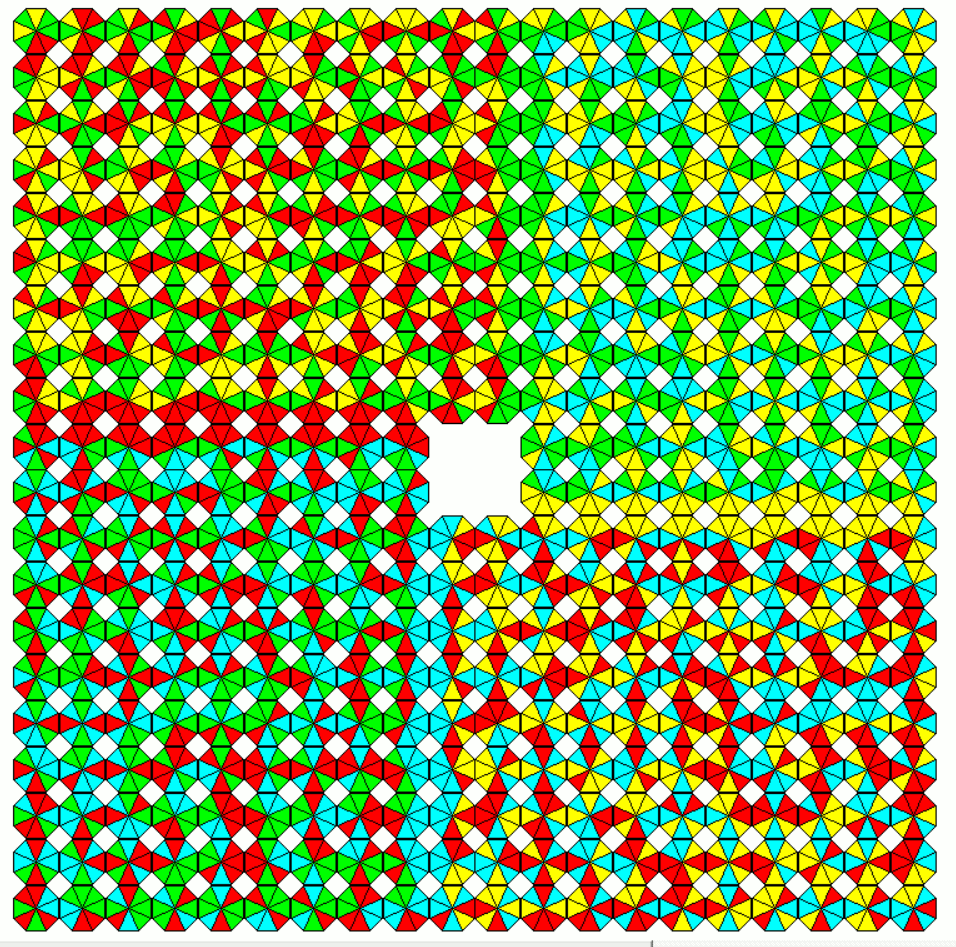## 4 Colors, Ratio of Color Frequencies is 2:3:3, One-sided

There are 840 one-sided pieces or four subsets of 210 pieces with one color missing. Solving rectangles of area 210 with additional border conditions yields for instance a 28x30 rectangle or a 29x29 square with a single hole.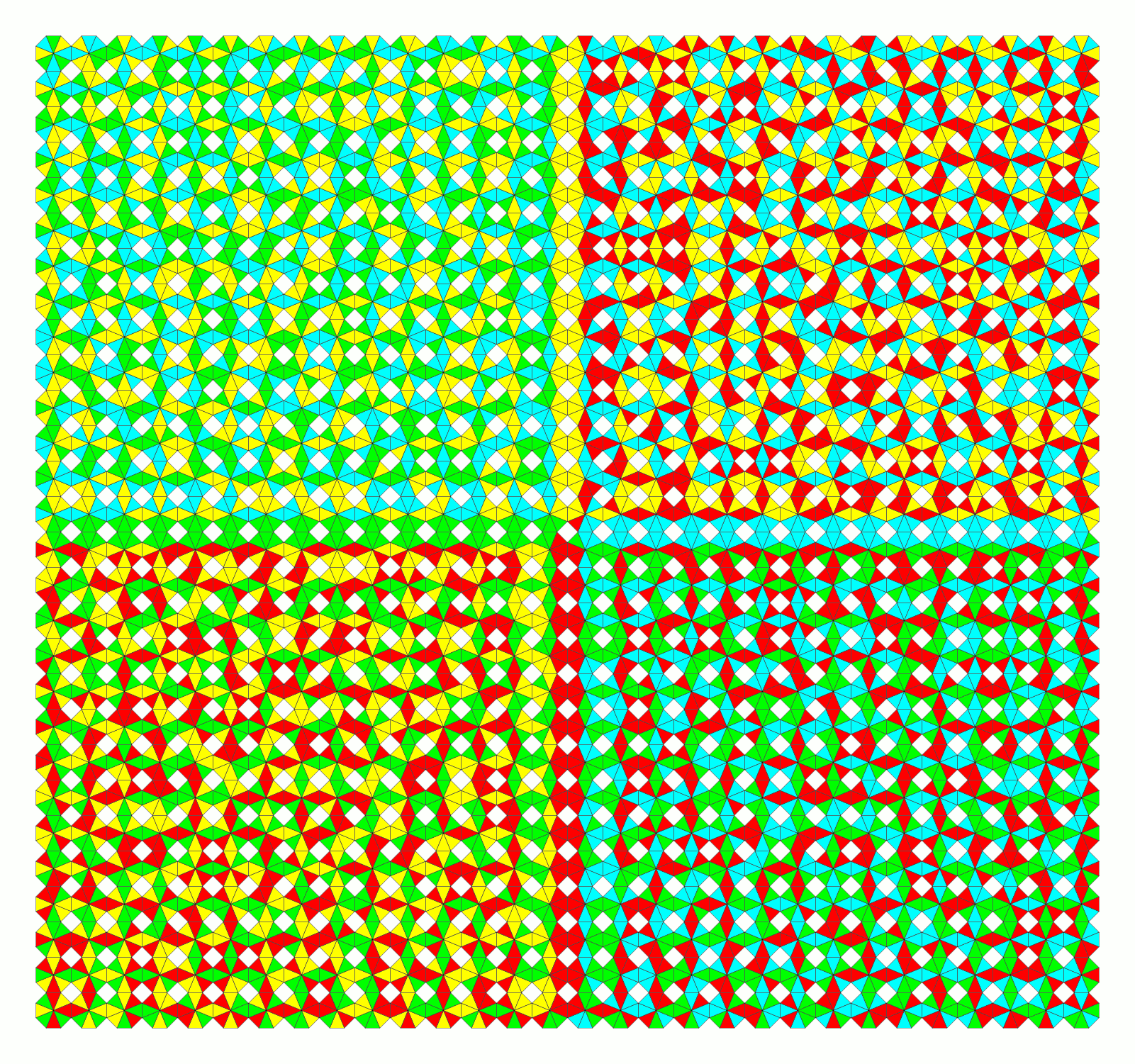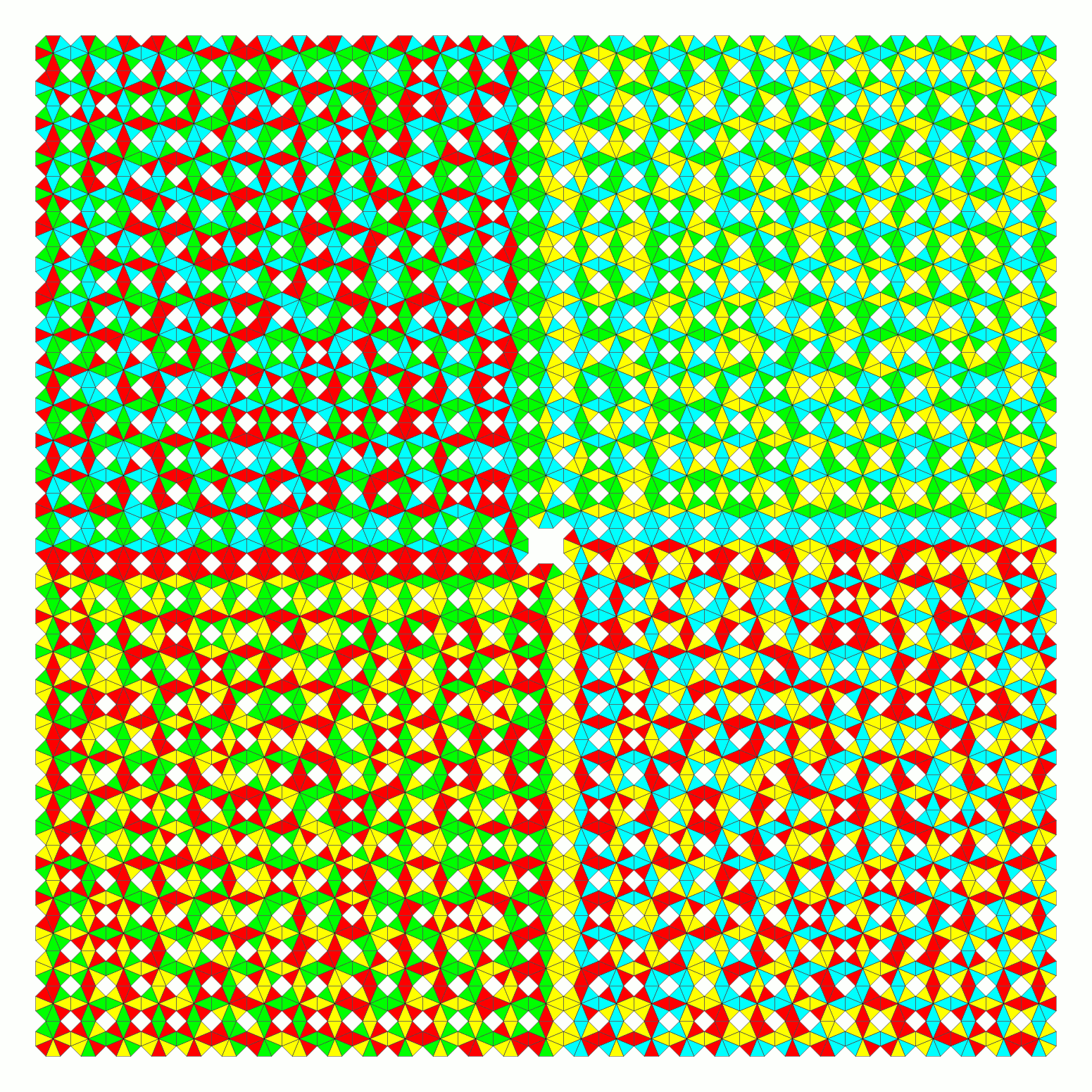## 4 Colors, Ratio of Color Frequencies is 2:3:3, Two-sided

Without splitting rectangles of size 8x57 and 12x38 can be covered by the 456 two-sided pieces.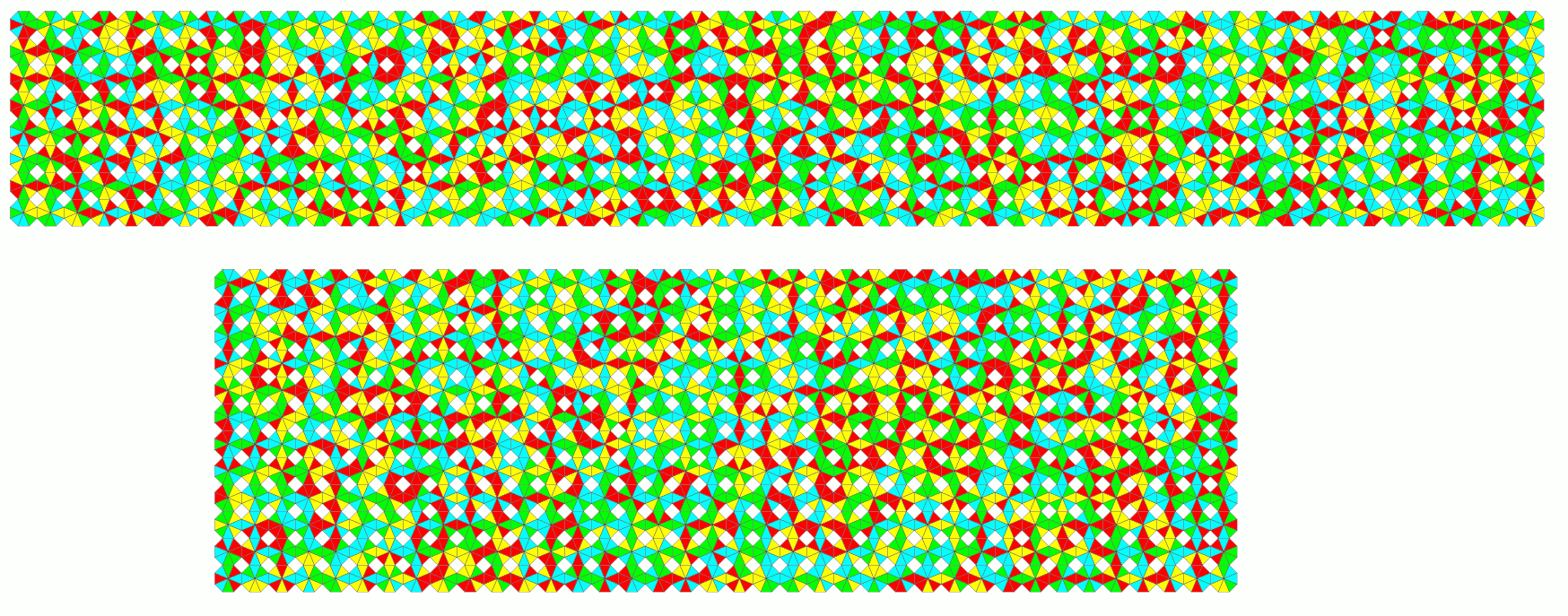For the 19x24 rectangle I used a different approach. I changed the color of a piece by replacing the colors 1,2,3,4 by 2,3,4,1, respectively and called this permutation a color shift. There are 114 sets of always four pieces which can be transformed into each other by color shifts. Using only one pieces of each set to solve a 19x6 rectangle three other rectangles are given by color shifts. If pieces at the border match we solved the large 19x24 rectangle. The upper left corners of the four rectangles are at columns 1, 7, 13 and 19. This way a solution was found almost immediately.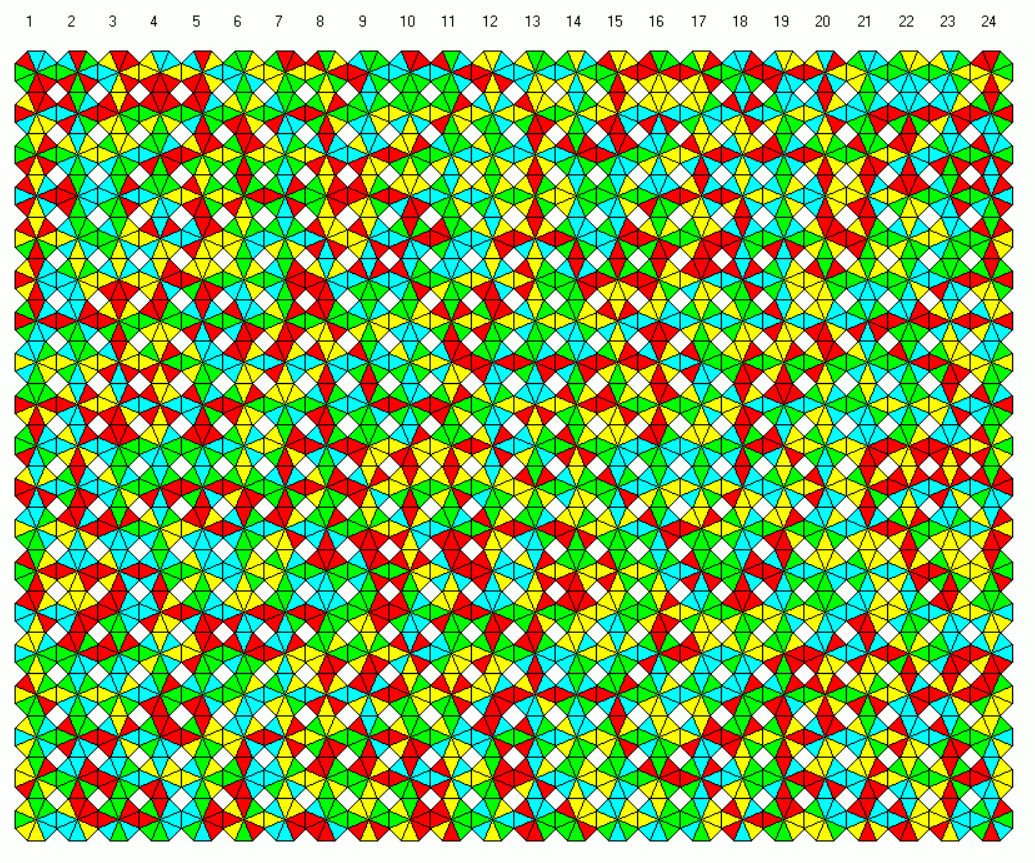## 4 Colors, Ratio of Color Frequencies is 1:3:4, One-sided

We have 840 one-sided pieces. These are as many pieces as pieces with 2:3:3 frequency ratio. Same problems can be solved by same methods. 10x84 and 28x30 rectangles are shown.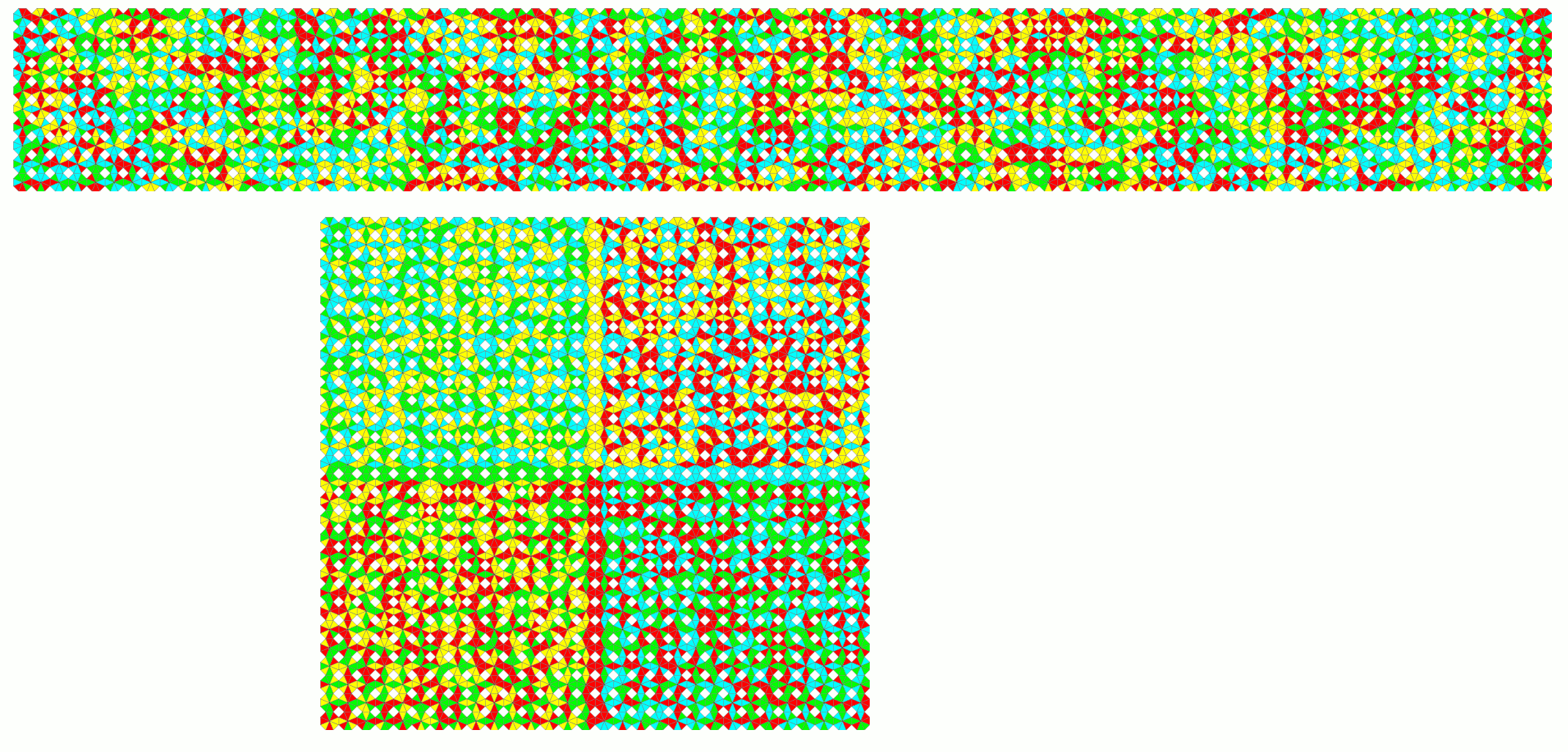## 4 Colors, Ratio of Color Frequencies is 1:3:4, Two-sided

Here are some constructions with the 456 two-sided pieces.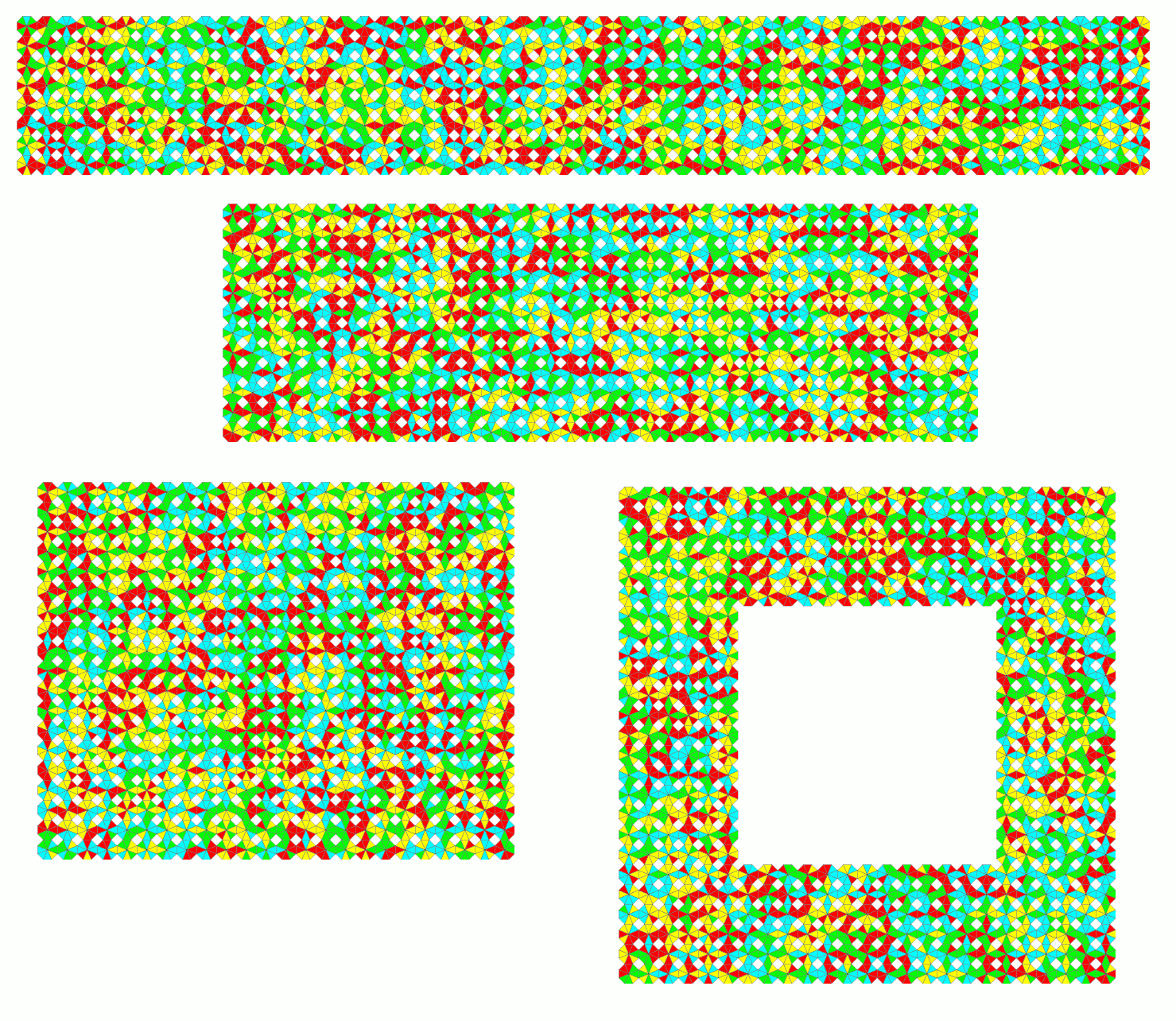Using the color shift method for the 19x24 rectangle a solution was found immediately.